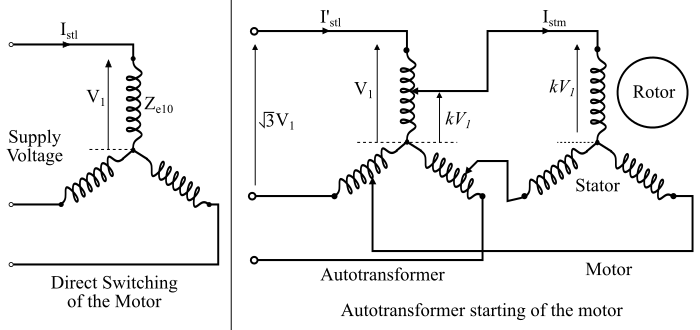# Working Principle of Autotransformer Starter

## Circuit and Working Principle of Autotransformer Starter

The circuit diagram of an autotransformer starter for starting a 3-phase induction motor is shown in the figure. The autotransformer starter can be used for starting both star and delta connected 3-phase induction motors. In this method, the starting current of the motor is limited by using a 3-phase autotransformer to decrease the initial applied voltage to the stator. The autotransformer is provided with a number of tappings to obtain the variable voltage.In the autotransformer starting method, the starter is connected to a particular tapping of the autotransformer to obtain the most suitable starting voltage. A changeover switch S is used to connect the autotransformer in the circuit for starting the motor.

When the handle (H) of the switch S is at the START position, the primary winding of the autotransformer is connected to supply line and the induction motor is connected to the secondary winding of the autotransformer. When the motor picks up the speed (about 80 % of the rated speed), then the handle H is thrown to the RUN position. Consequently, the autotransformer is isolated from the circuit and the motor is now directly connected to the supply line and gets its rated voltage. The handle is held in the RUN position by the under-voltage relay. When the supply voltage fails or falls below a certain value, then the handle is released and comes back to the OFF position. To provide the overload protection to the motor, a thermal overload relay is used in the motor circuit.

## Theory of Autotransformer Starter

The figure below shows the circuit connections for the direct switching of the motor and the autotransformer starting of the motor.When direct switching of the motor to the supply line −

Let,

𝑍𝑒10 = Per phase equivalent impedance of motor referred to stator at standstill

𝑉1 = Supply voltage per phase

Thus, when the full supply voltage per phase (V1) is applied to the motor with direct switching, then the starting line current drawn by the motor from the supply line is given by,

$$\mathrm{𝐼_{𝑠𝑡𝑙} =\frac{𝑉_{1}}{𝑍_{𝑒10}}… (1)}$$

Now, with autotransformer starting, if a tapping of autotransformer with transformation ratio kis used, then the voltage available across the secondary winding terminals of the autotransformer (i.e., the voltage per phase across the motor) is kV1. Hence, the starting current of the motor with autotransformer starting is given by,

$$\mathrm{𝐼_{𝑠𝑡𝑚} =\frac{𝑘𝑉_{1}}{𝑍_{𝑒10}}… (2)}$$

If the no-load current of the transformer is neglected, then, the ratio of currents in a transformer is inversely proportional to the voltage ratio, i.e.,

$$\mathrm{\frac{𝐼_{1}}{𝐼_{2}}=\frac{𝑉_{2}}{𝑉_{1}}}$$

$$\mathrm{\Rightarrow\:𝑉_{1}𝐼_{1} = 𝑉_{2}𝐼_{2}}$$

Thus,

$$\mathrm{𝑉_{1}{𝐼^{′}_{𝑠𝑡𝑙}} = 𝑘𝑉_{1}𝐼_{𝑠𝑡𝑚}}$$

Where, ${𝐼^{′}_{𝑠𝑡𝑙}}$ is the line current drawn from the supply by the autotransformer.

$$\mathrm{\Rightarrow 𝐼^{′}_{𝑠𝑡𝑙} = 𝑘𝐼_{𝑠𝑡𝑚} … (3)}$$

Substituting the value of 𝐼𝑠𝑡𝑚 from eqn. (2) in eq. (3), we get,

$$\mathrm{ 𝐼^{′}_{𝑠𝑡𝑙}=𝑘(\frac{𝑘𝑉_{1}}{𝑍_{𝑒10}})=\frac{𝑘^{2}𝑉_{1}}{𝑍_{𝑒10}}… (4)}$$

Therefore,

$$\mathrm{\frac{Starting\:current\:with \:autotransformer\:starter\:(𝐼′_{𝑠𝑡𝑙} )}{Starting\:current\:with\:direct\:switching \:(𝐼_{𝑠𝑡𝑙 })}=\frac{\frac{𝑘^{2}𝑉_{1}}{𝑍_{𝑒10}}}{\frac{𝑉_{1}}{𝑍_{𝑒10}}}= 𝑘^{2}}$$

$$\mathrm{\:therefore;\: Starting \:current\: with \:\:autotransformer\: starter= 𝑘^2\: × \:Starting \:current \:with \:direct \:switching … (5)}$$

Since the torque developed in an induction motor is directly proportional to the square of the applied voltage. Thus, the torque with direct switching of the motor is,

$$\mathrm{\tau_{𝑠𝑡𝑑} \propto 𝑉_{1}^{2} … (6)}$$

And, the torque developed with the autotransformer starter is,

$$\mathrm{\tau_{𝑠𝑡𝑎} \propto 𝑘^2 𝑉_{1}^{2} … (7)}$$

Hence,

$$\mathrm{\frac{Starting\:torque \:with\: autotransformer \:starter (\tau_{𝑠𝑡𝑎})}{Starting \:torque \:with \:direct \:switching (𝐼_{𝑠𝑡𝑑})}=\frac{𝑘^2𝑉_{1}^{2}}{𝑉_{1}^{2}} = 𝑘^2}$$

$$\mathrm{\therefore\:Starting\: torque\: with \:autotransformer\: starter\:=\:𝑘^2 × Starting \:torque \:with \:direct \:switching … (8)}$$

From the eqns. (5) and (8), it is clear that with an autotransformer starter, the starting current of the motor drawn from the main supply line and the starting torque are reduced to k2 times of their corresponding values with the direct switching starting of the motor

Updated on: 27-Aug-2021

8K+ Views